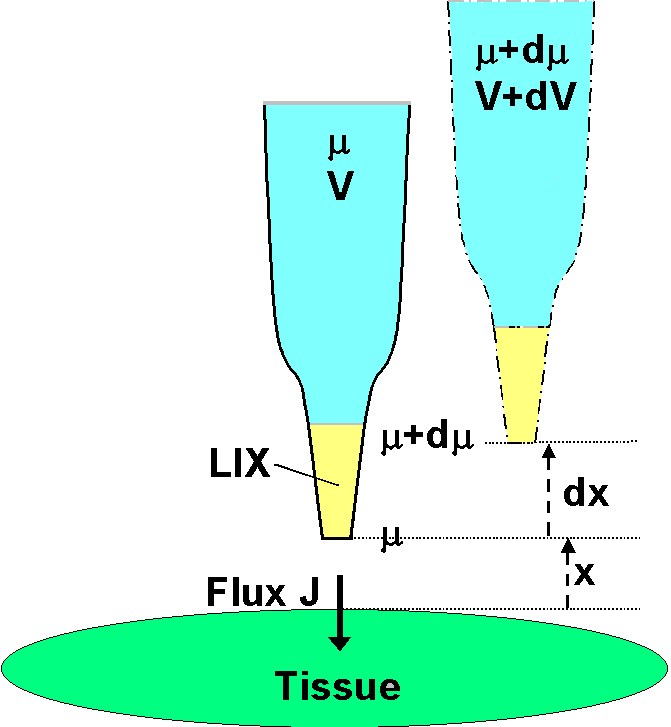# The MIFETM system  for non-invasive measurement of specific fluxes in solution near living plant or animal tissueHome
UTas Biophysics Lab
MIFE user group

MIFE theory
ion flux theory
multivalent ion mobility
ionic mobility values
neutral molecule flux
Methodological isues
H+ flux in buffered media

Hardware
amplifier
microscope
manipulator
System requirements

Software
analysis

Univ of Tas
Eppendorf NP2

## Principles and basic theory of microelectrode ion flux measurement

Chemicals in solution move under the influence of chemical forces of diffusion directed towards lower concentration regions. Ions, which are charged, also experience electrical forces if an electric field is present as well. The movement of an ion in solution can be described in terms of these chemical and electrical driving forces and other parameters of the ion and solution. It can be shown that the net flux of an ion, typically measured in units of nmol m-2 s-1, may be found from a measurement of the change in voltage of an ion selective microelectrode that is moved through a small known distance in the solution. This technique allows non-invasive measurement of net ion fluxes through a tissue boundary with resolution of 10 seconds in time and 20 micrometer in position. A suitable microscope is used to observe the microelectrodes and the tissue near which they are moved.

In the diagram a microelectrode, whose tip is filled with the liquid ion exchanger LIX, is initially at a distance x from the tissue into which ions are moving with a net flux J.It is assumed that there is no bulk solution flow, so that ionic movement in the solution (regardless of the membrane transport processes) is solely by diffusion under the influence of electric and chemical forces in solution. It is also assumed that the measurement is close to the surface and that the ionic movement is normal to the surface. The electrochemical potential in the solution at the distance x is µ (joules mol-1). Because the LIX allows free passage of the ion in question (but no others), the electrochemical potential of the ion inside the electrode is also µ. The chemical component of µ inside the electrode is fixed by the concentration of the filling solution; the electrical component is given by zFV , where V (volts) is measured by an electrometer connected via suitable half cells to the electrode solution and to a reference electrode some distance away in the bath solution. The ion's valence is z and F is the Faraday number. The microelectrode is now moved slowly away (not to disturb the solution) through a small distance dx. (It is shown offset in the diagram for clarity only.) At this new position in solution the electrochemical potential is µ + dµ. It is the same inside the electrode, but only the electrical component there has changed, and the measured voltage is now V + dV.

Basic electrochemical theory (See Newman, 2001) shows that the net ionic flux J is given in terms of the ion concentration c (mol m-3), the mobility of the ion u (speed per unit force, m s-1 per newton mol-1), and the force per mole which is the electrochemical potential gradient (dµ/dx). Thus J = c u (dµ/dx). But dµ is the same inside the electrode as in the bath solution, and in the electrode dµ = zFdV because the concentration inside is fixed. Hence the flux may be written J = c u z F(dV/dx). The concentration is known, or is adequately measured by the value of V when the electrode has been calibrated in standard solutions. For the ion, u and z are known constants, although for multi-valent ions u depends on z. The electrometer measures dV as the electrode is moved through the chosen distance dx. In practice, the LIX is not ideal, so the electrode is calibrated to find its actual "Nernst slope" (which depends on the valence).
When this is incorporated into the above flux equation, it becomes:
J = c u F(58/Nernst slope)(dV/dx).
This is the basic equation for ion fluxes. It is the basis of all implementations of the theory for ion flux measurement.

The theory can also be expressed in terms of the the diffusion coefficient D ( = u R T) for the ion instead of the mobility u.
For cylindrical geometry (e.g. a root surface) the radius of the cylinder (r) should be taken into account. This is done by replacing dx in the implementation of the equation above by
dx = r2 [1/(r+x) - 1/(r+x+dx)].
For spherical geometry (e.g.  a protoplast)
dx = r ln[(r+x+dx)/(r+x)].

The fluxes of neutral molecules, eg O2 and CO2, can also be measured using the technique.  An example is Pang et al  (2006).
In buffered media, part of the hydrogen ion (proton) flux is carried by protonated buffer molecules.
These and the many practical qualifications and limitations and methodological issues, are discussed in the literature, and particularly in the definitive review in the January 2001 Plant, Cell & Environment.
There is an ultimate limitation on the sensitivity of the technique, which is set by the thermal electronic noise in the high resistance of the ion selective liquid ion exchanger (LIX) in the micropipette tip. This theoretical limit is discussed in Ryan et al. (1990). That and other references to work using the technique are available.

Maintained by Ian Newman. Date . © University of Tasman